NEET  >  Short & Long Answer Questions: Mechanical Properties of Fluids - 2

# Short & Long Answer Questions: Mechanical Properties of Fluids - 2 - Notes | Study Physics Class 11 - NEET

 1 Crore+ students have signed up on EduRev. Have you?

Q.51 Hotter liquids move faster than colder ones. Why?

Answer: The viscosity or the internal force of friction of a liquid decreases with the increase in temperature. Hence hotter liquids move faster than colder ones.
Q.52 Why oils of different viscosity are used in different seasons?

Answer: Due to the rise of temperature in summer, the viscosity of oil decreases. Hence more viscous oils are used in summer than in winter.
Q.53 Why machine parts are jammed in the winter?

Answer: In winter season, the coefficient of viscosity of the lubricating oil gets increased due to fall in temperature. As a result of this, oil becomes more thick and therefore various machine parts are jammed.
Q.54 One flask contains glycerine and other contains water. Both are stirred vigorously and kept on the table. Which liquid will come rest to earlier than the other?

Answer: As the coefficient of viscosity of glycerine is greater than that of water, so the relative motion between different layers of glycerine is more strongly opposed compared to water. Hence glycerine comes to rest earlier than water.
Q.55 Why should the lubricant oil be of high viscosity?
Lubricant oil is used to reduce the dry friction between various machine parts. Due to high viscosity, lubricant oil sticks to the machine parts and cannot be squeezed out during the working of the machine.
Q.56 Why does an object entering the earth's atmosphere at high velocity catch fire?

Answer: When an object enters the earth's atmosphere at high velocity, its motion is strongly opposed by the viscous drag of air. As a result, the kinetic energy the object gets converted into heat energy. The heat produced may be so large that the object catches fire.
Q.57 The velocity of water in a river is less the bank and large in the middle. Why?

Answer: Due to large adhesive force between the water stream and the bank of the river, the velocity water is very small near the bank. It increases towards of middle of the river due to decrease in the adhesive force.
Q.58 Which fall faster - big rain drops or small rain drops?
The terminal velocity of rain drops υ=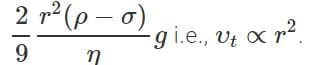Hence big drops fall faster.
Q.59 Why rain drops falling under gravity do not gain very high velocity?

Answer: When the rain drops fall freely under gravity, their motion is opposed by the viscous drag of air. They attain terminal velocity when the viscous force due to air becomes equal to the weight of the drops. Terminal velocity, υ∝r2 As radii of rain drops are small, so they do not acquire very high velocity.
Q.60 Why dust generally settles down in closed room?

Answer: Dust particles are spheres of small radii. After acquiring the terminal velocity, they start falling through air with uniform speed. As the terminal velocity for small dust particles will be very small, they will settle down in a closed room after some time.
Q.61 Why do clouds seen floating in the sky?

Answer: We know that terminal velocity of a spherical body falling through a viscous medium is directly proportional to the square of its radius. Hence terminal velocity of a small drop of water is very small. The small drops of water acquire this terminal velocity much before reaching the earth, and are seen to float in the sky in the form of clouds.
Q.62 Explain why parachute is invariably used, while jumping from an aeroplane.

Answer: A parachute experiences a large viscous force due to its huge structure. Hence, it descends through the air with a very small velocity and the person using the parachute does not get hurt.
Q.63 Fog particles appear suspended in the atmosphere. Give reason.

Answer: Fog particles have very small sizes. So their terminal velocity (υt∝r2) through air is very small. They appear suspended in the atmosphere.
Q.64 The sides of a horizontal pipe carrying dirty water get dirty. Why?

Answer: While flowing through the horizontal pipe, the velocity of dirty water is maximum along the axis of the pipe and minimum near the walls of the pipe. The viscous force opposes the relative motion between adjacent layers. Due to this, the dirt particles move outwards and get struck on the walls of the pipe. This makes the pipe dirty.
Q.65 Why two streamlines cannot cross each other?

Answer: If two streamlines cross each other, there will be two directions of flow at the point of intersection which is impossible.
Q.66 What happens when the velocity of a liquid becomes greater than its critical velocity?

Answer: The flow of liquid changes from streamline to turbulent.
Q.67 What happens to the external energy maintaining the flow of a liquid when the flow becomes turbulent?

Answer: In turbulent flow of a liquid, most of the external energy maintaining the flow is used in setting up the eddies in the liquid.
Q.68 Why does the velocity increase when water flowing in a broader pipe enters a narrow pipe?

Answer: This is due to equation of continuity: a1υ1=a2υ2 AS  a2<a1, so υ21
Q.69 Why still water runs deep?

Answer: In case of a deep water, the cross-sectional area, through which it flows, is quite large. Hence according to equation of continuity, its velocity is small and therefore it appears to be still.
Q.70 Why it is dangerous to stand near the edge of the platform when a fast train is crossing it?

Answer: When a fast train crosses the platform, the air dragged along with the train also moves with a high velocity. In accordance with Bernouilli's equation, the pressure in the region of high velocity gets decreased. If a person stands near the edge of the platform he may be pushed towards the train due to high pressures outside.
Q.71 Why two boats moving in parallel directions close to each other get attracted?

Answer: When the two boats come closer to each other, the velocity of water between the narrow gap increases and so pressure decreases in accordance with Bernouilli's equation. The pressure on the outer surfaces of both the row boats becomes greater than the pressure in the gap. Therefore, the two boats are pulled towards each other.
Q.72 Why does the speed of a liquid increase and its pressure decrease, when the liquid passes through a narrow constriction in a pipe?

Answer: When the liquid passes through a narrow constriction, its velocity increases (υ∝1/a) in accordance with the equation of continuity. The Bernouilli's equation for the horizontal flow is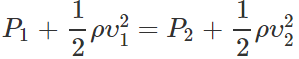As the velocity is high at the narrow constriction, so the pressure is low.
Q.73 Why does a flag flutter, when strong winds are blowing on a certain day?

Answer: When strong winds blow over the top of the flag, the kinetic energy of the wind at the top is more is more and hence pressure decreases. Due to difference in pressure above and below the flag, the flag flutters.
Q.74 On which principle does a carburetor work?

Answer: Carburettor is that chamber of an internal combustion engine in which air is mixed with petrol vapours. Air is allowed to enter through a nozzle with high velocity. The pressure is lowered at that point and petrol is sucked up into the chamber. The petrol vaporize quickly. The mixed vapours of petrol and air are then fed into the cylinder of internal combustion engine.
Q.75 Roofs of the huts are blown up during stormy days. Why?

Answer: The velocity of the wind is high above the roof. So the pressure is low in accordance with Bernouilli's principle. But the pressure below the roof is the atmospheric pressure which is high. So the roof is blown up.
Q.76 When air is blown between two balls suspended close to each other, they are attracts towards each other. Why?

Answer: When air is blown between the two balls, the velocity is increased and hence pressure is decrease (Benoulli's principle). On the outer sides of the balls, the pressure is high and hence the two balls get attracted.
Q.77 An aeroplane runs for some distance the runway before taking off. Why?

Answer: Generally, air does not strike an aeroplane with a large velocity. To get the lift, the aeroplane runs for some distance on the runway before taking off. Due to its special shape, the velocity of air above the plane increase and hence pressure decreases. The aeroplane gets uplift.
Q.78 The accumulation of snow on the aeroplane may reduce the lift. Explain.
Due to the accumulation of snow on the wings of the aeroplane, the shape of the wings no longer remains that of the aeroplane. This reduces the path difference and hence the velocity difference between the layer of air on the two sides of the wings. Hence the pressure difference on the two sides of the wings is reduced. This reduces the uplift on the aeroplane.
Q.79 Why bullets are given cylindrical shape?

Answer: The magnus effect is absent if the spinning cylinder is moving linearly in the direction parallel to spin axis. That is why the bullets are made cylindrical instead of spherical. They do not deviate from the linear path.
Q.80 According to Bernoulli's theorem, the pressure of water in a horizontal pipe of uniform diameter should remain constant. But actually it goes on decreasing with the increase in length of the pipe. Why?

Answer: Bernoulli's theorem is valid only for non- viscous liquids. But water is a viscous liquid. A part of the pressure energy of water is used in doing work against the viscous force. So the pressure of water decreases.
Q.81 What is the effect on the equilibrium of a physical balance when air is blown below one pan?

Answer: Due to increase in velocity of air below the pan, the pressure decreases. So the pan goes down.
Q.82 In case of an emergency, a vacuum brake is used to stop the train. How does this brake work?

Answer: Steam at high pressure is allowed to enter the cylinder of the vacuum brake. Due to high velocity, the pressure decreases in accordance with Bernoulli's principle. The reduction of pressure lifts up the piston. This in turn lifts up the brake.
Q.83 Is Bernoulli's theorem valid for viscous liquid?

Answer: No, it should be modified to take into account the work done against viscous drag.
Q.84 Two cylindrical vessels placed on a horizontal table contain water and mercury respectively up to the same heights. There is a small hole in the walls of each of the vessels at half the height of liquids in the vessels. Find out the ratio of the velocities of efflux of water and mercury from the holes. Which of the two jets of liquid will fall at a greater distance on the table from the vessel? Relative density of mercury with respect to water= 13.6.

Answer: The velocity of efflux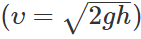is independent of density of liquid. So, both the jets of liquid will fall at the same distance.
Q.85 Bernoulli?s theorem holds for incompressible, non-viscous fluids. How is this relationship changed when the viscosity of the fluid is not negligible?

Answer: If the viscosity of the fluid is not negligible, a part of the mechanical energy of the fluid is spent in doing work against forces of viscosity. So the total energy: P+ρgh+1/2 ρυ2 of the fluid goes on decreasing along the direction of flow of the fluid.
Q.86 Why are the cars and aeroplanes given streamline shape?

Answer: Cars and aeroplanes are given streamline shape to minimize the backward drag of atmosphere.
Q.87 Why a glass rod coated with wax does not become wet when dipped in water?

Answer: The reason is that force of adhesion between water and wax molecules is smaller than force of cohesion between water molecules.
Q.88 Mercury does not cling to glass. Why?

Answer: The force of adhesion between mercury and glass is smaller than the force of cohesion between the mercury molecules.

Q.89 Why is it not possible to separate two pieces of paper joined by glue or gum?

Answer: The adhesive force between the molecules of glue and paper is much more than the cohesive force between the glue molecules. As a result, the two papers stick together with a large force and cannot be separated.
Q.90 Why does the free surface a liquid behave like an elastic stretched membrane?

Answer: The liquid molecules on the surface experience a downward force. So they have greater potential energy. In order to have minimum energy, the free surface tends to contract to minimum area and hence behaves like an elastic stretched membrane.

Q.91 Why does mercury collect itself into drops when placed on a clean glass plate?

Answer: The adhesive force between mercury and glass molecules is less than the cohesive force between mercury molecules. So instead of sticking to glass, mercury collects itself into drops.
Q.92 How dental plate clinges to the roof of the mouth?

Answer: The dental plate is made exactly parallel to the roof of the mouth at all the points. A small amount of saliva gets enclosed between dental plate and the roof of the mouth. Due to the force of adhesion between the saliva and dental plate, the plate clinges to the mouth.
Q.93 What shape does a liquid take when it weighs nothing?

Answer: When a liquid weighs nothing, surface tension is the only force acting on it. Due to surface tension, the liquid surface tends to occupy minimum surface area. For a given volume, the surface area of a sphere is minimum, so the liquid assumes spherical shape.
Q.94 One can form a fairly large vertical film of soap solution but not of pure water. Why?

Answer: This is because the surface tension of soap solution is much smaller than that of pure water. When we try to make a vertical film of pure water, it breaks due to the high surface tension of water.
Q.95 Small insects can move about on the surface of water. Why?

Answer: Due to surface tension, the free surface of water behaves like stretched elastic membrane which is able to support the small weight of insects and they can move on the surface of water.

Q.96 Why ends of a glass tube become rounded on heating?

Answer: When glass is heated, it melts to a liquid. The surface of this liquid tends to have a minimum area. Now, for a given volume, the area of the surface of a sphere is minimum. This is why the ends of a glass tube become rounded on heating.
Q.97 Antiseptics have low surface tension. Why?

Answer: Low surface tension helps the antiseptic to spread over large area over the wounds.

Q.98 Why hot soup tastes better than cold soup?

Answer: Hot soup has comparatively less surface tension than cold soup. So it spreads over larger area of the tongue and tastes better than cold soup.
Q.99 Oil spreads over the surface of water whereas water does not spread over the surface of oil. Why?

Answer: The surface tension of the water is more than that of oil. Therefore, when oil is poured over water, the greater value of surface tension of water pulls oil in all directions, and as such it spreads on the water. On the other hand, when water is poured over oil, it does not spread over it because the surface tension of oil being less than that of water, it is not able to pull water over it.
Q.100 Why does a stream of water from a faucet become narrow as it falls?

Answer: This is due to surface tension. The molecules of water come close to each other due to surface tension and surface area tends to be minimum making the stream narrow.

Q.101 Why do the hair of a shaving brush cling together when taking out of water?

Answer: When the brush is taken out of water, thin water film is formed at the tips of the hair. It contracts due to surface tension and so the hair cling together.
Q.102 A needle floats on the surface of pure water but goes down when detergent is added to water. Why?

Answer: Due to addition of detergent, the surface tension and hence the reaction of surface tension decreases. Hence the needle sinks due to smaller upward reaction.
Q.103 Why it becomes easier to spray the water in which some soap is dissolved?

Answer: When soap is dissolved in water, the surface tension of water decreases and so less energy is needed for spraying the water i.e., spraying of water in which soap is dissolved becomes easier.
Q.104 The clothes are better cleaned with hot water than with cold water. Why?

Answer: Surface tension decreases with the increase of temperature. Lesser the surface tension, more is the wetting (and hence the washing) power of water.
Q.105 How does soap help us to remove dirt better in washing clothes?

Answer: With the addition of soap, the surface tension of water decreases. The decrease in surface tension results in greater wetting and hence washing power.
Q.106 A oil drop on a hot cup of soup spreads over when the temperature of the soup falls. Why?

Answer: The surface tension of hot water is less than that of oil and hence oil drop does not spread over hot water. When water is cooled, its surface tension decreases. At low temperature, the surface tension of water becomes greater than that of oil and hence oil drop starts spreading over it.
Q.107  Glass marbles are made by heating the end of a glass rod until drops of molten glass fall. Explain.

Answer: When drops of molten glass fall freely, they are in state of weightlessness. As only the force of surface tension acts on them, so they acquire spherical shape which on solidification become glass marbles.
Q.108 Oil is sprinkled on sea waves to calm them. Why?

Answer: When oil is sprinkled, the breeze spreads the oil on the sea-water in its own direction. The surface tension of sea-water (without oil) is greater than oily water. Hence the water without oil pulls the oily water against the direction of breeze, and the sea waves become calm.
Q.109 A tiny liquid drop is spherical but a larger drop has oval shape. Why?

Answer: In the case of a tiny drop, the force of surface tension is large compared to its weight, so the drop has spherical shape. A large drop has oval shape because the force of gravity (weight) exceeds the force of surface tension.
Q.110 Why does a small piece of camphor dance about on the water surface?
Due to its irregular shape, the camphor piece dissolves more rapidly at some points than at others. Where it dissolves, the surface tension of water is reduced. As the force of surface tension reduces by different amounts at different points of the camphor piece, a resultant force acts on it which makes it dance about on water surface.
Q.111 The addition of flux to tin mat soldering easy. Why?

Answer: The addition of flux reduces surface tension of the molten tin. This helps it spread easily over the area of soldering.
Q.112 The paints and lubricating oils have low surface tension. Why?

Answer: The paints and lubricating oils having low surface tension can spread over a large surface area.
Q.113 Why are the droplets of mercury when brought in contact pulled together to from a bigger drop? Also state with reason whether the temperature of bigger drop will be the same, or more, or less than the temperature of the smaller drops?

Answer: Due to surface tension, liquid drops tend to have minimum surface area. When mercury droplets are brought in contact, they form one drop thereby decreasing the surface area. Due to decrease in surface, surface energy is lost by the bigger drop which appears as heat. So its temperature increases.
Q.114  The angle of contact for a solid and a liquid is less than 90. Will the liquid wet the solid? Will the liquid rise in the capillary made of that solid?

Answer: The liquid will wet the solid and will rise in the capillary tube made of that solid.
Q.115 Write down formula for excess pressure inside (i) a liquid drop (ii) a soap bubble.

Answer: (i) For a liquid drop: p= 2σ/R (ii) For a soap bubble: p= 4σ/R where σ is surface tension.
Q.116 Why excess pressure in a soap bubble is twice the excess pressure of a liquid drop of the same radius?

Answer: A soap bubble has two free surfaces, one internal and another external; whereas liquid drop has only one outer free surface.
Q.117 Two soap bubbles of unequal sizes are blown at the ends of a capillary tube. Which one will grow at the expense of the other and what does it show?

Answer: The bigger one will grow at the expense of the smaller one. This is because excess pressure is inversely proportional to radius and air flows from higher pressure to lower pressure.
Q.118 What is the importance of (i) wetting agents used by dyers, and (ii) water proofing agents?

Answer: (i) They are added to decrease the angle of contact between the fabric and the dye so that the dye may penetrate well. (ii) They are used to increase the angle of contact between the fabric and water to prevent the water from penetrating the cloth.
Q.119 Teflon is coated on the surface of non-sticking pans. Why?

Answer: When the surface of a pan is coated with teflon, the angle of contact between the pan and the oil used for the frying purpose becomes obtuse. Thus the frying pan becomes non-sticking.

Q.120 What makes water-proof rain coat water-proof?

Answer: The angle of contact between water and the material of the raincoat is obtuse. So the rainy water does not wet the raincoat i.e., the raincoat is water-proof.
Q.121 How does the ploughing of fields help in preservation of moisture in the soil?

Answer: This is done to break the tiny capillaries through which water can rise and finally evaporate. The ploughing of field helps the solid to retain the moisture.
Q.122  How does the cotton wick in an oil-filled lamp keep on burning?

Answer: The narrow spaces between the threads of the wick serve as capillary tubes through which oil keeps on rising due to capillary action.
Q.123 Why sand is drier than clay?

Answer: Due to narrow pores in clay, water rises in the clay due to capillary action and keeps it damp. Practically no pores or capillaries exist in sand and hence water cannot rise in sand. So sand is drier than clay.
Q.124 Why undergarments are usually made of cotton?

Answer: Cotton threads have large number of capillaries between them. These capillaries help to absorb sweat from the surface of the body due to capillarity action.
Q.125 A piece of chalk immersed in water emits bubbles in all directions. Why?

Answer: A chalk piece has a large number of capillaries. As it is immersed in water, water rises due to capillary action. The air present in the chalk is expelled out in the form of bubbles in all directions.
Q.126 Why new earthen pots keep water cooler than the old one?

Answer: Due to capillary action, water oozes out of the pores of the new earthen pot. This water takes latent heat from the pot to evaporate and so the water in the pot gets cooled. In old pots, most of the capillaries are blocked. So the cooling is not so effective.
Q.127 Why is the tip of the nib of a pen split?

Answer: The split in the tip of the nib acts as a capillary tube. The ink rises up in the nib due to capillary action. Hence we are able to write with the pen.
Q.128 Water rises in a capillary tube, whereas mercury falls in the same tube. Why?

Answer: In a capillary tube, a liquid rises to a height h given by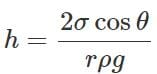For water, θ is acute, cosθ is positive and hence h is positive. So water rises in the capillary tube. For mercury, θ is obtuse, cosθ is negative and hence h is negative. So mercury gets depressed in the capillary tube.
Q.129 Why is it difficult to make mercury enter a fine thermometer tube?

Answer: The meniscus of mercury in a glass tube is convex. Since there exists an excess pressure (p=2σ/r) on the concave side of the curved liquid surface, the pressure below the meniscus is greater than the atmospheric pressure. This excess pressure has a very large value for a tube of fine bore. As the pressure of mercury inside the tube is greater than that outside it, mercury instead of entering into the tube tends to flow out.
Q.130 Water gets depressed in a glass tube whose inner surface is coated with wax. Why?

Answer: The angle of contact between water and wax is obtuse. So cosθ is negative and hence capillary rise: h=2σcosθ/rρg is also negative. That is why water gets depressed.
Q.131 If a capillary tube is immersed at first in cold water and then in hot water, the height of capillary is smaller in the second case. Why?

Answer: The height up to which a liquid rises in a capillary tube is given by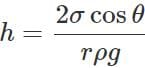The surface tension (σ) of hot water is less than that of cold water. Moreover, capillary tube expands in hot water, so its radius r increases. So capillary rise h is smaller in hot water than in cold water.
Q.132 If a capillary tube is put in water in a state of weightlessness how will the rise of water in a capillary tube be different to one observed under normal conditions?

Answer: In normal conditions, when the force of surface tension (due to which water rises in capillary) becomes equal to the weight of the water column raised in the tube, water stops rising. In the state of weightlessness, the effective weight of water column raised is zero. Hence water will rise up to the other end of the capillary, however long the capillary is. Water will not overflow, its surface will become flat (its radius of curvature will become infinity)
Q.133 How is the rise of liquid affected, if the top of the capillary tube is closed?

Answer: As the liquid rises in the capillary tube, the air gets compressed between the top end of the tube and the liquid meniscus. The compressed air opposes the rise of liquid due to surface tension. The liquid rises till the two opposing forces just balance each other. Hence if the top end of the capillary tube is closed, the liquid rises to a smaller height.
Q.134 A 20 cm long capillary tube is dipped in water. The water rises up to 8 cm. If the entire arrangement is put in a freely falling elevator, what will be the length of water column in the capillary tube?

Answer: In the freely falling elevator, the entire arrangement is in a state of weightlessness, i.e., g= 0. So water will rise in the tube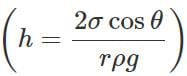to fill the entire 20 cm length of the tube.
Q.135 Spherical balls of radii R are falling in a viscous fluid of viscosity η with a velocity υ. How does the retarding viscous force acting on a spherical ball depend on R and υ?

Answer: F=6πηRυi.e., retarding viscous force is directly proportional to both R and υ.
Q.136 Why do air bubbles in a liquid move in upward direction?

Answer: The density of air bubble is less than that of liquid. Initially, the resultant of up thrust and the viscous force is greater than the weight of the air bubble. So, the air bubble experiences a net upward force. Then bubble soon attains a terminal velocity in the upward direction.
Q.137 Explain why some oils spread on water, when others float as drops.

Answer: If the surface tension of oil is less than that of water, then it spreads on water. If the surface tension of oil is more than that of water, then it floats as drops on water.
Q.138 What happens when a capillary tube of insufficient length is dipped in a liquid?

Answer:  When a capillary tube of insufficient length is dipped in a liquid, the liquid rises to the top. The radius of curvature of the concave meniscus increases till the pressure on its concave side becomes equal to the pressure exerted by the liquid column of insufficient length. But the liquid does not overflow.
Q.139 Problem 1. Explain why : (i) A balloon filled with helium does not rise in air indefinitely but halts after a certain height (Neglect winds). (ii) The force required by a man to raise his limbs immersed in water is smaller than the force for the same movement in air.

Answer: (i) A balloon filled with helium goes on rising in air so long as the weight of the air displaced by it (i.e., up thrust) is greater than the weight of filled balloon. We know that the density of air decreases with height. Therefore, the balloon halts after attaining a height at which density of air is such that the weight of air displaced just equals the weight of filled balloon.

(ii) Water exerts much more up thrust on the limbs of man than air. So the net weight of limbs in water is much less than that in air. Hence the force required by a man to raise his limbs immersed in water is smaller than the force for the same movement in air.
Q.140 What height of water column produces the same pressure as a 760 mm high column of Hg?

Answer: Pressure exerted by h height of water column =Pressure exerted by 760 mm of Hg column ∴h×1000×9.8=0.760×13.6×103×9.8 [P=hρg] or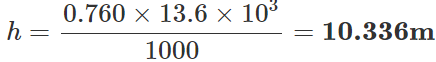Q.141 A small ball of mass m and density ρ is dropped in a viscous liquid of density ρ. After some time, the ball falls with a constant velocity. Calculate the viscous force on the ball.

Answer: Volume of the ball, V= m/ρ Mass of the liquid displaced, m′=Vρ0=m/ρ .ρ0 When the body falls with a constant velocity, Viscous force = Effective weight of the ball F=Weight of the ball ? Up thrust =mg−m′g or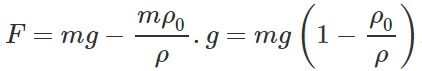Q.142 A tank filled with fresh water has hole in its bottom and water is flowing out of it. If the size of the hole is increased what will be the change in: (a) Volume of water flowing out per second? (b) Velocity of the out coming water? (c) If in the above tank, the fresh water is replaced by sea water, will the velocity of out coming water change?

Answer: (a) The volume of water flowing out per sec will increase as its volume depends directly on the size of hole.

(b) The velocity of outflow of water remains unchanged because it depends upon the height of water level and is independent of the size of the hole.

(c) No, though the density of sea water is more than that of fresh water, but the velocity of outflow of water is independent of the density of water.
Q.143 In a bottle of narrow neck, water is poured with the help of an inclined glass rod. Why?

Answer: If water is directly poured into a bottle of narrow neck, the stream of water blocks the neck due to the pressure of inside air and the strong force of adhesion between glass and water. If a small part of the glass rod is placed inside the bottle and water is poured along the length of the rod outside the bottle, water molecules cling to the glass rod and the force of gravity pulls down these molecules into the bottle.
Q.144 The excess pressure inside a soap bubble is thrice the excess pressure inside a second soap bubble. What is the ratio between the volume of the first and the second bubble?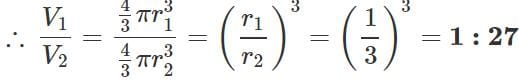Q.145 The viscous force 'F' acting on a body of radius 'r' moving with a velocity ' υ' in a medium of coefficient of viscosity ?η? is given by F=6πηrυ. Check the correctness of the formula.

∴ Dimensions of LHS = Dimensions of RHS.

Hence the given formula for viscous force F is dimensionally correct.
Q.146  A tornado consists of rapidly whirling air vortex. Why is the pressure always much lower in the centre than at the outside? How does this condition account for the destructive power of tornado?

Answer: The angular velocity of air in a tornado increases as it goes towards the centre. As the air moves towards the centre, its moment of inertia (I) decreases and to conserve angular momentum (L=Iω), the angular velocity co increases. Because of the enormous increase in velocity of inner layers, the air pressure at the centre reduces greatly in accordance with Bernoulli's theorem. This sudden reduction in pressure may prove highly disastrous both for life and property in the vicinity of a tornado.

The document Short & Long Answer Questions: Mechanical Properties of Fluids - 2 - Notes | Study Physics Class 11 - NEET is a part of the NEET Course Physics Class 11.
All you need of NEET at this link: NEET

## Physics Class 11

127 videos|464 docs|210 tests

## Physics Class 11

127 videos|464 docs|210 tests

### How to Prepare for NEET

Read our guide to prepare for NEET which is created by Toppers & the best Teachers

Track your progress, build streaks, highlight & save important lessons and more!

,

,

,

,

,

,

,

,

,

,

,

,

,

,

,

,

,

,

,

,

,

;